## OSDM Demo Tutorial – Making of ‘Darkness – Oh Xee A Moron, Part 2′

Categories: EDUCATION, OSDM, TUTORIAL
Tags: ,
Comments: No Comments
Published on: September 11, 2014

Hi Folks,
Here is another Oldskool Demomaker Demo Tutorial of my popular production “Darkness, Oh Xee A Moron, Part 2“.

You can download the final Windows Executable here (Roy-Demo-Darkness.zip)

Also, all elements, such as 3D Objects, Images, Fonts and the Preference .INI Configuration file are also available for download here (Darkness.zip)

# Watch Video Capture

To get an idea how the final product looks like, without touching OSDM or downloading anything, watch the video capture that I created below.

Backup URL to Video on vimeo (http://www.vimeo.com/14096272).

Backup Links to Vimeo and YouTube, as well as additional download options (e.G. a Full Screen Version of the Demo) are available at my official demo release page.

# OSDM Settings Screen-By-Screen

## Main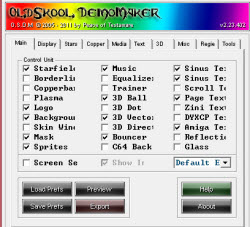Enabled Effects: Starfield Music Sinus Text 16 Sinus Text 32 3D Ball Page Text Logo Background 3D Vector Amiga Text Mask Bouncer Sprites Screen Setup: Off Default Export

## Display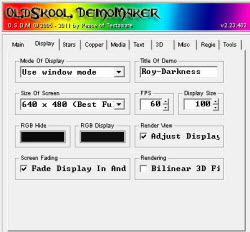Mode: Use window mode (full screen would shift 3d objects to invalid positions) Title: Roy-Darkness Size of Screen: 640×480 FPS: 60 (different value would mess up timing) Display Size: 100 RGB Hide & Display: Black Render View, Adjust Display: On Fade Display In And Out: On Bilinear 3D Filter: Off

## Stars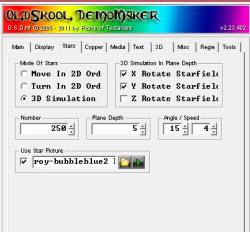Mode: 3D Simulation X Rotate Starfield: On Y Rotate Starfield: On Z Rotate Starfield: Off Number: 250 Plane Depth: 5 Angle: 15, Speed: 4 Use Star Picture: On (roy-bubbleblue2 bw.png)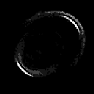## Logo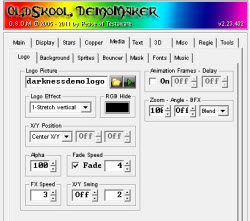Picture: darknessdemologo.png Animation: off Effect: 1-Stretch vertical RGB Hide: Black Zoom: 100, Angle: Off, BFX: Blend X/Y Position: Center X/Y Alpha: 100, Fade: On, Speed: 4 FX Speed: 3, X/Y Swing: Off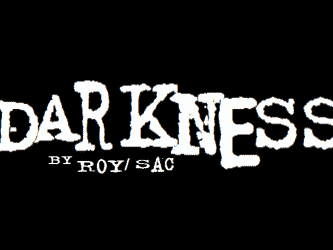## Background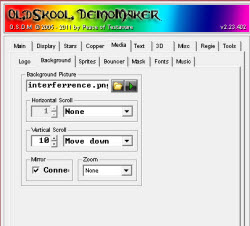Picture: interferrence.png Horizontal Scroll: None Vertical Scroll: 10, Move down Mirror: On Zoom: None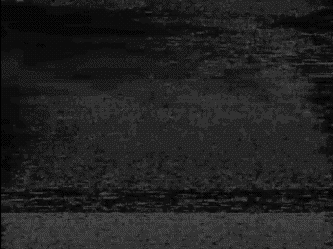## Bouncer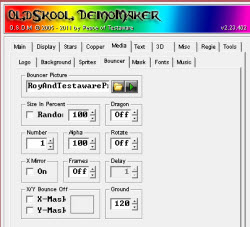Picture: RoyAndTestawareProd.png Size; Random: Off, 100%, Dragon: Off Number: 1, Alpha: 100, Rotate: Off X Mirror: Off, Frames: Off X-Mask & Y-Mask: Off Ground: 120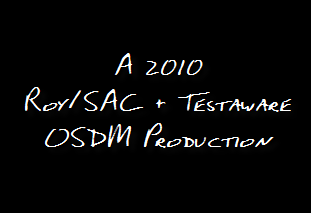## Mask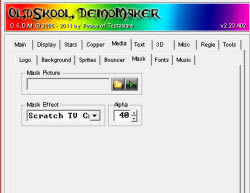Picture: None Mask Effect: Scratch TV Crumble Alpha: 40

## Fonts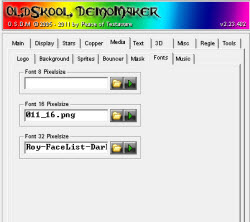16 Pixels: 011_16.png (for Sinus 16 Scroller)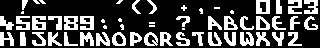32 Pixels: Roy-FaceList-Darkness-Font32x32-outlined.png (for Sinus 32 Scroller & Page Text)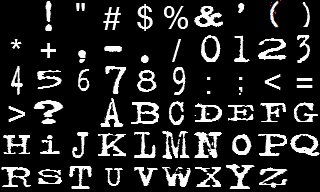## Music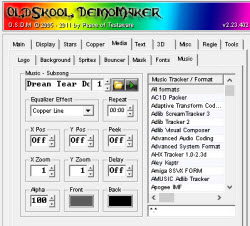Song: Drean Tear Down by Teque-Trauma.xm Subsong: 1

## Sprites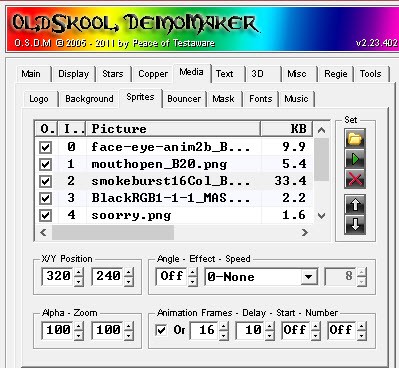Initial Sprites Settings

#NameX/Y-PosAngEffSpeed AlphaZoomAnimFrmsDelayStartNum
0face-eye-anim2b_B10.png320/240 Off 100100 On1090OffOff
1mouthopen_B20.png320/240 Off 100100 On2035OffOff
2smokeburst16Col_B16.png320/240 Off 100100 On1610
3BlackRGB1-1-1_MASK.png320/240 Off 100100 Off
4soorry.png320/240 73-Move-left6 100100 Off
5darkness-note.png320/240 Off 100100 Off
6faceeyesopen2_B9.png320/180 Off 10090 On935
7visitroysaccom.png320/240 Off 100100 Off

 0 – face-eye-anim2b_B10.png 1 – mouthopen_B20.png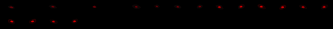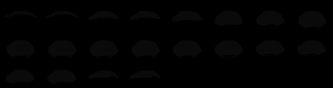2 – smokeburst16Col_B16.png 3 – BlackRGB1-1-1_MASK.png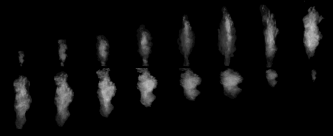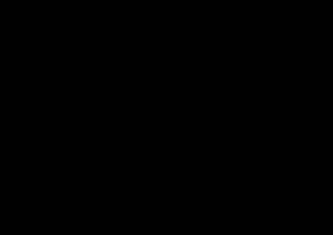4 – soorry.png 5 – darkness-note.png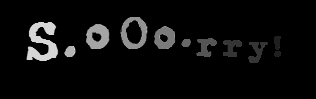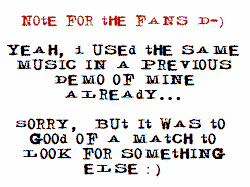6 – faceeyesopen2_B9.png 7 – visitroysaccom.png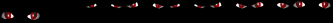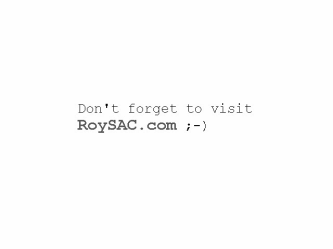## Sinus Text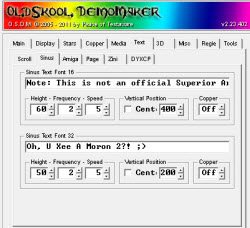Text Font 16: “Note: This is not an official Superior Art Creations production! None of my OSDM productions are. “ Height: 60; Frequency: 2; Speed: 5 Vertical Pos; Center: Off, 400 Copper: Off Text Font 32: “Oh, U Xee A Moron 2?! 😉 “ Height: 50; Frequency: 2; Speed: 5 Vertical Pos; Center: Off, 200 Copper: Off

## Amiga Text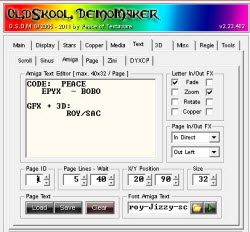Letter In/Out FX In: Fade Out: Zoom Page In FX: In Direct Page Out FX: Out Left Page Lines: 5; Wait: 40 X/Y Position: 20/90 Size: 32 Font Picture: roy-Jizzy-scenealphabet-font-OSDM-32×32-grayscale3.png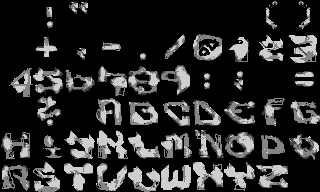Text Page 1 ```CODE: PEACE EPYX - BOBO GFX + 3D: ROY/SAC``` Page 2 ```[ [ [ [ [``` Page 3 ```MUSIC: "DREAM TEAR DOWN" BY TEQUE/TRAUMA``` Page 4 ```[ [ [ [ [```

## Page Text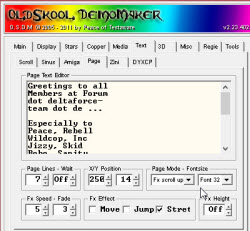Page Lines: 7, Wait: Off X/Y Position: 250/14 Page Mode: FX scroll up Font Size: 32 FX Speed: 5 Fade: 3 Fx Effect: Stretch Fx Height: Off Text: ```"Greetings to all Members at Forum dot deltaforce- team dot de ... Especially to Peace, Rebell Wildcop, Inc Jizzy, Skid Bobo, Sanity Dragon, Marie Genom, Synth Twilight and Janer. Cheers! Roy/SAC in 2010! Signing off. "```

## 3D Vector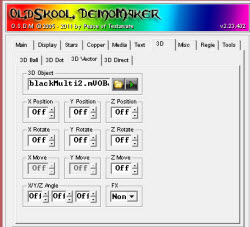Object: blackMulti2.mVOBJ X/Y/Z Position: Off X/Y/Z Rotate: Off X/Y/Z Move: Off X/Y/Z Angle: Off FX: None blackMulti2.mVOBJ consists of 4 individual vector objects 0…3 0 – Roy-Face20comb-Black-Closed.vobj 1 – sphere.vobj 2 – Roy-2TORUS-Black.vobj 3 – Roy-3TORUS-Black.vobj Build-in Tool to create Multi-Vector Object File from individual .VOBJ objects.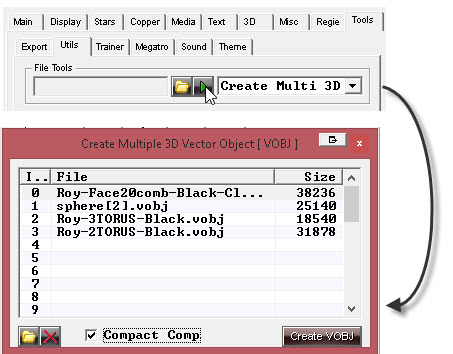0 – Roy-Face20comb-Black-Closed.vobj 1 – sphere.vobj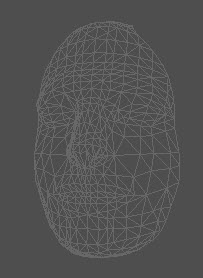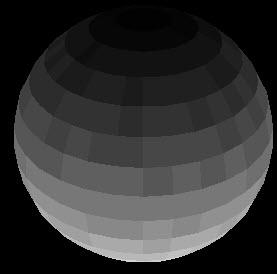2 – Roy-2TORUS-Black.vobj 3 – Roy-3TORUS-Black.vobj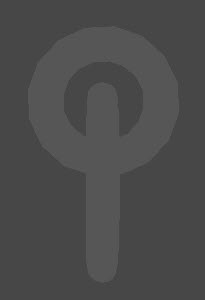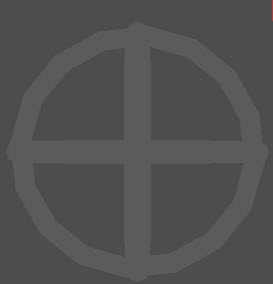## 3D Ball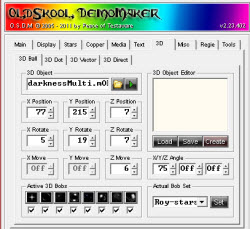Object: darknessMulti.mOBJ
X/Y/Z Position: 77/215/7
X/Y/Z Rotation: 5/19/7
X/Y/Z Move: Off/Off/6
X/Y/Z Angle: 75/Off/Off

Bobs Images

 0 1 2 3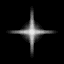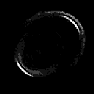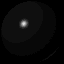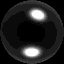4 5 6 7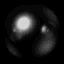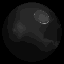darknessMulti.mOBJ consists of 6 individual 3D Ball objects 0-5

0 – rings3.obj
1 – rings4.obj
2 – roy-5rings-wobbler-vectormania.obj
3 – roy-CCU-Synth FullscreenCube.OBJ
4 – Roy-wall-1st Level Squared.obj
5 – Roy-wall-TowerObj.obj

Build-in Tool to create Multi-3DBall Object File from individual .OBJ objects.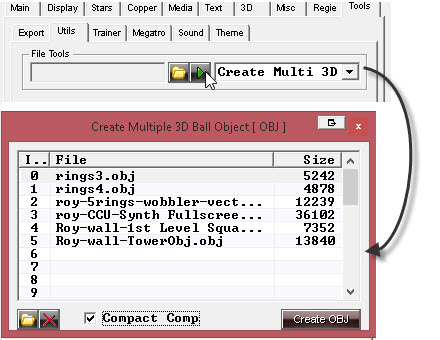0 – rings3.obj 1 – rings4.obj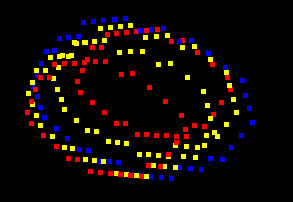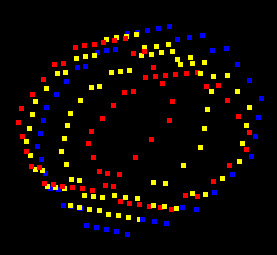2 – roy-5rings-wobbler-vectormania.obj 3 – roy-CCU-Synth FullscreenCube.OBJ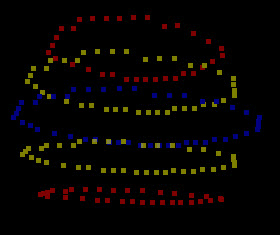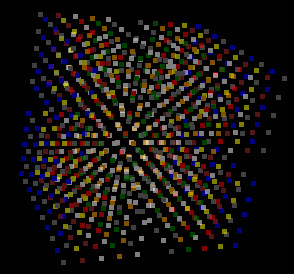4 – Roy-wall-1st Level Squared.obj 5 – Roy-wall-TowerObj.obj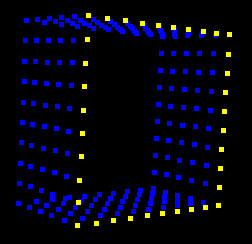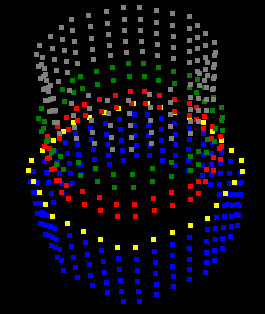## Script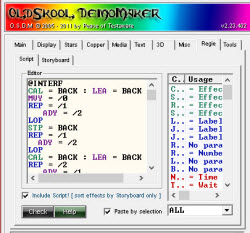Include Script!: On Script Source code shown further down

## Story BoardLine: Off, Fade: off, Speed: 1 Initial order from top to bottom. Only enabled effects are listed. D – Starfield G – 3D Vector Object I – 3D Ball Object L – Sinus Text 16 M – Sinus Text 32 N – Page Text H – Bouncer O – Mask X – Sprite W – Amiga Text J – Logo B – Background

## Export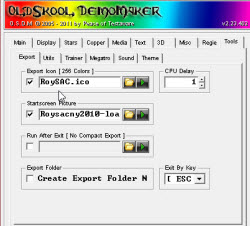Export Icon: RoySAC.ico
CPU Delay: 1 Exit by Key: [ ESC ] Startscreen Picture: Roysacny2010-loading2.png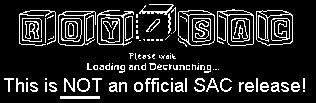# Script Code

```@INTERF
CAL = BACK : LEA = BACK
MVY = /0
REP = /1 : ADY = /2 : LOP
STP = BACK
REP = /1 : ADY = /2 : LOP
CAL = BACK : LEA = BACK
REP = /2 : ADY = /1 : LOP
STP = BACK
@END

;windowed/fs
;also see sprite init

@INIT3D
LEA = 3DVECTOR
IDN = /0
;Windowed
MVX = -600 : MVY = -350
;Full Screen
;MVX = 0 : ;MVY = -50
MVZ = -250
@END

@ADJBALL
;off for windowed
;enable for Full screen
; ADX = 100 : ADY = -150
@END

PSH
FPS = 60
CAL = MUSIC+SPRITE
LEA = SPRITE
IDN = 7 : MVA = 0
IDN = 6 : MVA = 0
;Windowed enable
;full screen disable
;next 1 line
ADX = 25 : ADY = -50
IDN = 5 : MVA = 255
CAL = DISPLAY

;-----------------
;Init
;-----------------
LEA = SPRITE
IDN = 0 : MVA = 0 : ADY = -100 : ADX = 150
IDN = 1 : MVA = 0 : MVX = 500 : MVY = 380 : MVS = 900
IDN = 2 : MVA = 0 : MVX = 500 : MVY = 320 : ADW = -20
IDN = 3 : MVA = 0 : MVW = 640 : MVH = 480
IDN = 4 : MVA = 0 : ADX = 120 : ADY = 140

;------------------
NOP = 4
LEA = SPRITE : IDN = 5
REP = 120 : ADA = -2 : LOP
MVA = 0
STP = SPRITE

;----------------
;End Note Display
;--------------
CAL = 3DVECTOR
LEA = 3DVECTOR : IDN = 0

;--------------
;Init 3D Vect
;--------------
@INIT3D = 0

MAX = 0 : MAY = 0 : AAZ = 0
ADY = 380 : ADZ = 280
BFX = 2 : NOP = 2
CAL = MASK

REP = 100 : ADY = -2 : ADZ = -2 : LOP
STP = MASK

@INTERF = 150,2,3

LEA = 3DVECTOR : IDN = 0

REP = 45 : AAX = 1 : ADY = -2 : ADZ = -2 : LOP
BFX = 4

@INTERF = 350,4,2

CAL = MASK+BOUNCE
LEA = BOUNCE : MVX = 500 : MVY = 360
LEA = 3DVECTOR : IDN = 0
REP = 45 : AAX = 1 : ADY = -2 : ADZ = -2 : LOP
STP = MASK
NOP = 1
MRY = -3 : NOP = 3
CAL = MASK : STP = BOUNCE
MRY = 4
REP = 150 : ADZ = 2 : ADX = -2 : LOP

@INTERF = 100,4,12

LEA = 3DVECTOR : IDN = 0
REP = 150 : ADZ = 2 : ADX = -2 : LOP
STP = MASK
CPW = 240 : CPX = 2 : MRY = -4
REP = 150
ADZ = -4 : ADX = 2
IFX > -400 : ADX = 1
LOP
CAL = LOGO : CAL = MASK
MRY = 3 : BFX = 1 : NOP = 1
STP = MASK
MRY = -3 : BFX = 4
REP = 150 :  ADZ = 3 : ADX = -3 : LOP
STP = LOGO : CAL = MASK
MRY = 0
REP = 90 : AAX = -1 : LOP
STP = MASK
CPX = 0

;---------------
; Cloning End
;---------------
ADX = 480
REP = 90 : AAX = 1 : LOP
@INTERF = 300,10,7
LEA = 3DVECTOR : IDN = 0
MRY = -3
REP = 200 : ADX = 2 : ADZ = -2 : LOP
MRY = 3 : NOP = 1

;---------------
;Eyes Blend in
;---------------
CAL = SPRITE
LEA = SPRITE : IDN = 0
REP = 200 : ADA = 1 : LOP
LEA = 3DVECTOR : IDN = 0 : MRY = 0

;---------------
;Mouth Open
;---------------
LEA = Sprite : IDN = 0 : MVS = 9999
IDN = 1 : MVS = 150 : MVA = 210 : NOP = 1 : MVS = 900

;-----------
;Smoke and Soorry
;------------
CAL = SINUS32 : LEA = SINUS32
MVZ = 105 : MVX = -325 : MVS = -3
LEA = SPRITE : IDN = 2
REP = 25
ADA = 5 : ADY = -2 : ADW = 1 : ADH = 2
LOP
REP = 25
ADA = -5 : ADY = -2 : ADW = -2 : ADH = -1
LOP
IDN = 1 : MVS = 150
REP = 200
IDN = 0 : ADA = -1
IDN = 1 : ADA = -1
IDN = 4 : ADA = 1
LOP
IDN = 0 : MVA = 0
IDN = 1 : MVA = 0
IDN = 4
REP = 20 : ADA = -10 : LOP
MVA = 0
STP = SPRITE
LEA = 3DVECTOR : IDN = 0
REP = 200 : ADX = 1 : LOP
STP = DISPLAY
REP = 100 : ADX = 2 : LOP
STP = SINUS32 : CAL = DISPLAY

;------------
;3D BALLS
; plus sphere
;------------
CAL = SPRITE+3DBALL
LEA = 3DBALL : IDN = 5
@ADJBALL
BFX = 1 : NOP = 1
@INTERF = 300,10,7
LEA = SPRITE : IDN = 3
REP = 150 : ADA = 1 : LOP

;--------------
;Sphere
;--------------
LEA = 3DVECTOR : IDN = 1
IDL = TRAINER
MVZ = 900 : ADY = 260
BFX = 1 : ARY = -30
MAX = 90 : MAY = -70
REP = 200
ADY = -2 : ADX = -3 : ADZ = -5
LOP
ARY = 15
LEA = 3DBALL : IDN = 5
ARY = -10
LEA = 3DVECTOR : IDN = 1
REP = 60
ADY = 2 : ADX = 1 : ADZ = 2
LOP
MRY = 3

;------------
;Object 5 - Tower
;--------------
LEA = 3DBALL : IDN = 5
ARY = -5 : NOP = 1
ARY = 5 : NOP = 1
ARY = 5 : NOP = 1
ARY = 5
CAL = LOGO
@INTERF = 400,15,5

;-------------
;Ball Bounce
;--------------
LEA = 3DVECTOR : IDN = 1
NOP = 3 : STP = LOGO
MRY = -15
REP = 50
ADX = -2 : ADZ = -1
LOP
MRY = -20 : MRX = 20
REP = 300
ADX = 1 : ADZ = 3
LOP
BFX = 4 :MRY = 15
CAL = MASK
REP = 200
ADX = -2 : ADZ = -4
LOP
STP = MASK
NOP = 2 : MRY = -15

;---------------
;Credits
;---------------
CAL = AMIGATEXT
REP = 10 : ADX = -10 : LOP
REP = 60 : ADX = 10 : LOP
NOP = 2
REP = 60 : ADX = -10 : LOP
LEA = AMIGATEXT : IDN = 2
LEA = 3DVECTOR : IDN = 1
REP = 60 : ADX = 10 : LOP
NOP = 2
REP = 50 : ADX = -10 : LOP
NOP = 1
STP = AMIGATEXT

;--------------
REP = 100 : ADY = -6 : LOP
STP = 3DBALL
@INTERF = 150,5,11

;---------------
; 3 Torus
;---------------
CAL = STARS
LEA = 3DVECTOR : IDN = 2
IDL = TRAINER

;---------------

BFX = 4 : ADY = 1000
BFX = 4 : ADX = 50
REP = 75 : ADY = -5 : LOP

;-------------
;Greetings
;-------------
CAL = PAGE
MRX = 0
REP = 15
ARY = 1
IFR = 1 : IDN = 2
IFR = 3 : IDN = 2
IFR = 2 : IDN = 2
LOP
MRY = 0 : MRZ = 0 : BFX = 2
LEA = SPRITE : IDN = 3
REP = 50 : ADA = -3 : LOP
MVA = 0
@INTERF = 50,2,2
LEA = 3DVECTOR : IDN = 2
REP = 200
IFR = 8 : ARX = 2
LOP
MRZ = 10 : NOP = 3
BFX = 3 : ARX = 10
CAL = MASK
REP = 200
ADX = 2 : ADY = 1
LOP
STP = MASK
REP = 30
ADY = 2 : ADX = 1
LOP
@INTERF = 100,4,8
LEA = 3DVECTOR : IDN = 2
REP = 150
ADX = -2 : ADY = -2
LOP
BFX = 1
REP = 50
ADX = -1 : ADY = 1
LOP
BFX = 4
@INTERF = 350,4,2
LEA = 3DVECTOR : IDN = 2
REP = 50
ADX = -1 : ADY = 1
LOP
ARX = -10 : NOP = 4 : ARY = 20
REP = 300 : ADX = 4 : LOP
STP = DISPLAY : NOP = 1
STP = PAGE+STARS

;---------------
;3DBalls Part
;--------------
CAL = 3DBALL

;--------------
;Object 2 - Wobbler
;--------------
LEA = 3DBALL : IDN = 2
BFX = 4 : ADX = 100 : ADY = 150
CAL = SPRITE
LEA = SPRITE : IDN = 3 : MVA = 150
CAL = DISPLAY
NOP = 4
REP = 75 : ADA = -2 : LOP
MVA = 0 : NOP = 2
@INTERF = 240,2,1

;--------------
;Object 1 - Rings 4
;--------------
LEA = 3DBALL : IDN = 1
ADX = -100 : ADY = 50
REP = 150
ADX = 2 : ADY = -1
LOP
REP = 180
ADX = -2 : ADY = 1
LOP
REP = 100
ADX = 1 : ADY = -1
LOP
REP = 80 : ADX = 1 : LOP
BFX = 2 : NOP = 2
@INTERF = 40,4,7

;--------------
;Object 0 - Rings 3
;--------------
LEA = 3DBALL : IDN = 0
NOP = 2
CAL = MASK
NOP = 1
STP = MASK
LEA = SPRITE : IDN = 3
REP = 100 : ADA = 1 : LOP

;--------------
;Object 4 - Square
;--------------
LEA = 3DBALL : IDN = 4
BFX = 4 : MRX = -10
REP = 150 : AAZ = -2 : LOP
CAL = MASK
REP = 150 : AAZ = -2 : LOP
STP = MASK
RGB = \$EEE : CLS = 2

;--------------
;Object 3 - Cube
;--------------
LEA = 3DBALL : IDN = 3
ADY = 100 : ARY = -10
REP = 20
ADZ = 20 : ADY = -3
LOP
ARY = -10 : NOP = 1
ARY = 5 : ARX = 5 : ARZ = 5 : NOP = 1
ARY = 5 : ARX = 5 : ARZ = 5 : NOP = 1
ARY = 5 : ARX = 5 : ARZ = 5 : NOP = 1
CAL = MASK
REP = 180
ADY = 3 : ADX = -3
LOP
STP = MASK
REP = 360
ADY = -3 : ADX = 3
LOP
CAL = STARS

;-------------
;2 torus
;--------------
LEA = 3DVECTOR : IDN = 3
ADZ = 100
REP = 315
ADX = -4 : ADZ = -1
LOP
MRZ = 15 : MRX = 10 : MRY = 25
LEA = SPRITE : IDN = 3
REP = 100 : ADA = -1 : LOP
MVA = 0
LEA = 3DVECTOR : IDN = 3
REP = 150 : ADX = 2 : LOP
CAL = MASK
REP = 75
ADX = -2 : ADY = -2
LOP
STP = MASK
BFX = 1
REP = 75
ADX = -2 : ADY = 2
LOP
CAL = MASK
REP = 75
ADX = 2 : ADY = 2
LOP
BFX = 4
STP = MASK
REP = 225 : ADY = -2 : LOP
CAL = LOGO : NOP = 4
STP = LOGO
REP = 300 : ADY = 4 : LOP

;------------
;Face Again
;------------
CAL = SINUS16
@INIT3D = 0
MRX = 0 : MRY = 0 : MRZ = 0 : ADX = 400 : ADY = 0
MAY = 0 : MAZ = 0 : MAX = 90 : MRY = 2
REP = 200 : ADX = -2 : LOP
MRY = -2 : NOP = 3 : MRY = 0

;-------------
; Eye Animation
;-------------
CAL = SPRITE
LEA = SPRITE : IDN = 6 : MVA = 255
STP = DISPLAY+SINUS16
LEA = 3DVECTOR : IDN = 0 : BFX = 1 : NOP = 1
STP = STARS+3DVECTOR

;----------------
; Vist RoySAC.Com
;----------------
CAL = DISPLAY
LEA = SPRITE
REP = 54
IDN = 6 : ADA = -4
IDN = 7 : ADA = 4
LOP
IDN = 6 : MVA = 0
IDN = 7 : MVA = 255
NOP = 2

;-------------
;Fade Out Music
;-------------
REP = 99 : ADV = -1 : LOP
NOP = 1

ESC : END
```

# Closing Notes

For more OSDM tutorials, tools, demos, intros and resources; visit the OSDM Section on my web site.

Cheers!

Carsten aka Roy/SAC

NOTE! I believe in the right for freedom of speech and personal opinion and are against censorship, so feel free to tell me what you think and let me and others hear your opinion on this subject, but please avoid using the f-word and s-word as much as you possibly can, because at the end of the day this blog exists for the purpose of useful exchanges of thoughts, ideas and opinions and not as a valve for your accumulated anger and frustration. Get a shrink for that! Thanks.

Welcome , today is Sunday, June 20, 2021